## Rendering an image with VTK

For exploring and visualizing various pocketing or area-clearing milling toolpath strategies I am thinking about reviving the "pixel-mowing" idea from 2007. This would be simply a bitmap with different color for stock remaining and already cleared area. It would be nice to log and/or plot the material removal rate (MRR), or the cutter engagement angle. Perhaps graph it next to the simulation, or create a simulated spindle-soundtrack which would e.g. have a pitch proportional to the inverse of the MRR. It should be possible to demonstrate how the MRR spikes at sharp corners with classical zigzag and offset pocketing strategies. The solution is some kind of HSM-strategy where the MRR is kept below a given limit at all times.
My plan is to play with maximally-inscribed-circles, which are readily available from the medial-axis, like Elber et. al (2006).

Anyway the first step is to render a bitmap in VTK, together with the tool/toolpath, and original pocket geometry: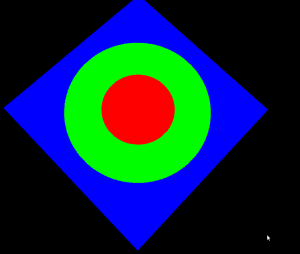```import vtk import math   vol = vtk.vtkImageData() vol.SetDimensions(512,512,1) vol.SetSpacing(1,1,1) vol.SetOrigin(0,0,0) vol.AllocateScalars() vol.SetNumberOfScalarComponents(3) vol.SetScalarTypeToUnsignedChar()   scalars = vtk.vtkCharArray() red = [255,0,0] blue= [0,0,255] green= [0,255,0] for n in range(512): for m in range(512): x = 512/2 -n y = 512/2 -m d = math.sqrt(x*x+y*y) if ( d < 100 ): col = red elif (d<200): col = green else: col = blue for c in range(3): scalars.InsertTuple1( n*(512*3) + m*3 +c, col[c] )   vol.GetPointData().SetScalars(scalars) vol.Update()   ia = vtk.vtkImageActor() ia.SetInput(vol) ia.InterpolateOff()   ren = vtk.vtkRenderer() ren.AddActor(ia) renWin = vtk.vtkRenderWindow() renWin.AddRenderer(ren)   iren = vtk.vtkRenderWindowInteractor() iren.SetRenderWindow(renWin) interactorstyle = iren.GetInteractorStyle() interactorstyle.SetCurrentStyleToTrackballCamera()   camera = vtk.vtkCamera() camera.SetClippingRange(0.01, 1000) camera.SetFocalPoint(255, 255, 0) camera.SetPosition(0, 0.1, 500) camera.SetViewAngle(50) camera.SetViewUp(1, 1, 0) ren.SetActiveCamera(camera) iren.Initialize() iren.Start()```

## Cutting down the octree

If you number the octants in an octree using a 1982 scheme called Gargantini-code, and store the codes for only the black nodes in the tree in a list then that's called a linear octree.

For quadtrees, there is a 1985 paper by Bauer with an algorithm for computing set-operations (intersection, union, difference) between two trees. Ten years later Jiang corrected a few mistakes and extended this algorithm to octrees. Neither paper is free of misprints, but by looking at both I seem to have arrived at set-operations which work.

Pushing lists with 10 000 or more elements back and forth between C++ and python is not very fast, and I'm now rendering each node (a cube) in a tree as a separate Cube-object in VTK, which isn't very efficient. The video shows depth 6 trees at the end, and here's a screen-capture of a depth 7 tree:This could be useful for making a cutting-simulator used for both verifying CAM-algorithms in opencamlib, and G-code produced by other programs. It's probably possible to hook into the EMC2interpreter and have it drive the tool in the simulation.

## Octree in python

Recursion and 'divide-and-conquer' must be two of the greatest ideas ever in computer science.

Threw together some code in python today for building an octree, given a function isInside() which the tree-builder evaluates to see if a node is inside or outside of the object. Nodes completely outside the interesting volume(here a sphere, for simplicity) are rendered grey. Nodes completely inside the object are coloured, green for low tree-depth, and red for high. Where the algorithm can't decide if the node is inside or outside we subdivide (until we reach a decision, or the maximum tree depth).

In the end our sphere-approximation looks something like this: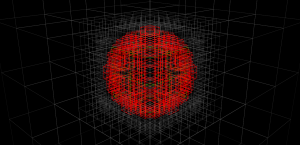These tests were done with a maximum tree depth of 6 or 7. If we imagine we want a 100 mm long part represented with 0.01 mm precision we will need about depth 14, or a subdivision into 16384 parts. That's impractical to render right now, but seems doable. In terms of memory-use, the octree is much better than a voxel-representation where space is uniformly subdivided into tiny voxels. The graph below shows how the number of nodes grows with tree-depth in the actual octree, versus a voxel-model (i.e. a complete octree). For large tree-depths there are orders-of-magnitude differences, and the octree only uses a number of nodes roughly proportional to the surface area of the part.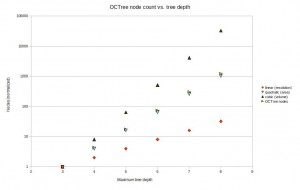Now, the next trick is to implement union (addition) and difference (subtraction) operations for octrees. Then you represent your stock material with one octree, and the tool-swept-volume of your machining operation with another octree -> lo and behold we have a cutting-simulator!

## Offset ellipse, part 2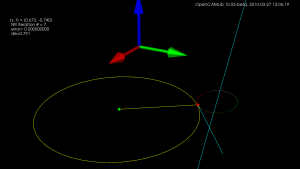More on the offset-ellipse calculation, which is related to contacting toroidal cutters against edges(lines). An ellipse aligned with the x- and y-axes, with axes a and b can be given in parametric form as (a*cos(theta) , b*sin(theta) ). The ellipse is shown as the dotted oval, in four different colours.

Now the sin() and cos() are a bit expensive the calculate every time you are running this algorithm, so we replace them with parameters (s,t) which are both in [-1,1] and constrain them so s^2 + t^2 = 1, i.e. s = cos(theta) and t=sin(theta). Points on the ellipse are calculated as (a*s, b*t).

Now we need a way of moving around our ellipse to find the one point we are seeking. At point (s,t) on the ellipse, for example the point with the red sphere in the picture, the tangent(shown as a cyan line) to the ellipse will be given by (-a*t, b*s). Instead of worrying about different quadrants in the (s,t) plane, and how the signs of s and t vary, I thought it would be simplest always to take a step in the direction of the tangent. That seems to work quite well, we update s (or t) with a new value according to the tangent, and then t (or s) is calculated from s^2+t^2=1, keeping the sign of t (or s) the same as it was before.

Now for the Newton-Rhapson search we also need a measure of the error, which in this case is the difference in the y-coordinate of the offset-ellipse point (shown as the green small sphere, and obviously calculated as the ellipse-point plus the offset-radius times a normal vector) and where we want that point. Then we just run the algorithm, always stepping either in the positive or negative direction of the tangent along the ellipse, until we reach the required precision (or max number of iterations).

Here's an animation which first shows moving around the ellipse, and then at the end a slowed-down Newton-Rhapson search which in reality converges to better than 8 decimal-places in just seven (7) iterations, but as the animation is slowed down it takes about 60-frames in the movie.

I wonder if all this should be done in Python for the general case too, where the axes of the ellipse are not parallel to the x- and y-axes, before embarking on the c++ version?

## Drop-cutter again

This is about my third rewrite of this fairly simple cam-algorithm where the cutter is dropped from above until it touches a triangle. It's now in C++ with Boost-python bindings and with visualization using vtk.

The cutter-location points are calculated by bringing the cutter into contact with the vertices of the triangle (green cl-points), the edges (red cl-points), and the facet (blue cl-points). Then the cl-point with the highest Z-value is selected as the final cutter location. At the white points we did not make contact with the triangle at all.

If you look closely enough, all surfaces in the world are made of triangles, even Tux:

Due to compression, the video might not show enough detail, so here's a screenshot: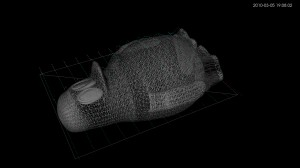I wonder if anyone is still interested in this stuff? Given enough time I would like to develop waterline-paths and an octree-based cutting simulator also. It would help if these algorithms were incorporated in a CAD-program, or someone would develop a GUI.

## VTK test

Tried to make the code from last time a bit clearer by splitting it into two files:

conversion to video again by first converting PNG to JPEG:

mogrify -format jpg -quality 97 *.png

And then encoding JPEGs into a movie:

mencoder mf://*.jpg -mf fps=25:type=jpg -ovc lavc -lavcopts vcodec=mpeg4 -ac copy -o output.avi -ffourcc DX50

## Learning VTK

I'm trying to learn how to render and animate things using VTK. This is the result of a python-script which outputs a series of PNG-frames. These are then converted to jpegs by this command:

mogrify -format jpg -quality 97 *.png

`mogrify -format jpg -quality 97 *.png`

and converted to a DIVX movie like this:
`mencoder mf://*.jpg -mf fps=25:type=jpg -ovc lavc -lavcopts vcodec=mpeg4 -ac copy -o output.avi -ffourcc DX50`

This should lead to the revival of my old Drop-Cutter code in the near future. This time it's going to be in C++, with Python bindings, and hopefully use OpenMP. Stay tuned.